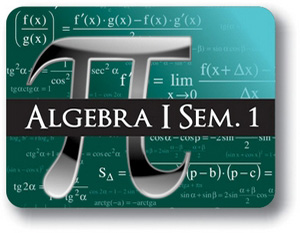Red Comet## Algebra I - Semester - 1

#### Course Price: \$285.00## Course Details:

This course provides an introduction to High School Algebra. Student learns about the history of algebra and the various mathematicians who have contributed to this field. The course introduces the student to variables and algebraic expressions and properties. Student learns to evaluate variable expressions and equations and solving linear equations. They learn about one-variable inequalities and understand how to graph them. The course then delves into two-variable linear equations and two-variable inequalities. Students study the Slope-intercept form and learn how to write and graph slope-intercept equations. They explore the Point-slope form and the Standard form. The course teaches students how to graph two-variable inequalities and about Absolute Value equations and inequalities.

Note: This course is not designed for ELL (English Language Learners) students. ELL students may enroll in this course ONLY if they have adequate mentor support at their home school and are able to fulfill all course requirements.

## Syllabus:

Section 1: Introduction & History of Algebra

• History of Algebra
• Basics of Algebra
• Writing Algebraic Expressions
• Combining like terms

Section 2: One-Variable Equations

• Evaluating Variable Expressions
• Solving One-Variable Linear Equations: One Step Solutions
• Solving One-Variable Linear Equations: Multi-Step Solutions
• Solving Linear Equations With Variables on Both Sides

Section 3: One-Variable Inequalities

• Introduction to Variable Inequalities
• Evaluating Variable Inequalities
• Graphing One-Variable Inequalities

Section 4: Two-Variable Linear Equations

• Introduction to Two-Variable Linear Equations
• Evaluating Multi-Variable Linear Equations
• Slope-intercept form
• Graphing Linear Equations
• Writing Slope-Intercept Equations
• Point-Slope Form
• Standard Form

Section 5: Two-Variable Inequalities

• Introduction to Two-Variable Inequalities
• Graphing Two-Variable Inequalities
• Absolute Value Equations
• Absolute Value Inequalities

### Accreditation & Approvals